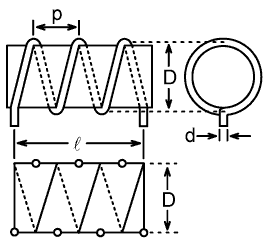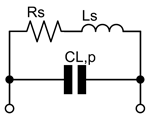```inductance calculator, by Serge Stroobandt, ON4AARound wire coil with dimensions and its current-sheet approximation

D
mm
Mean diameter of the air core coil, measured from wire centre to wire centre

N

Number of turns

ℓ
mm
Lenght of the coil, measured from the connecting wires centre to centre

d
mm
Wire or tubing diameter

Cu, annealed
Cu, hard-drawn
Ag
Al
Plating material

ρ
nΩ·m
Plating conductivity

μr

Plating permeability

f
MHz
Design frequency

INTERMEDIATE RESULTS:

p
mm
Winding pitch

Φ

Proximity factor according to empirical Medhurst data[2,3]

Deff
mm
Effective coil diameter according to Stroobandt (see below)

Correction factors:

kL

Field non-uniformity correction factor according to Lundin[2,4]

ks

Round wire self-inductance correction factor according to Rosa[2,5,6]

km

Round wire mutual-inductance correction factor according to Grover and Knight[2,7]

Wire:

ℓwire, phys
mm
Physical wire length

ℓwire, eff
mm
Effective wire length

δi
µm
Skin depth at design frequency

Sheath helix waveguide mode

ψ
°
Effective pitch angle

β
Axial propagation factor of n=0 sheath helix waveguide mode at design frequency[1,8]

Zc
Ω
Characteristic impedance of n=0 sheath helix waveguide mode at design frequency

Effective circuit equivalent at design frequencyLeff,s
µH
Effective series inductance at design frequency from Corum & Corum's sheath helix waveguide formula, corrected for field non-uniformity and round wire[1,2,4-7]

Xeff,s
Ω
Effective series reactance of round wire coil at design frequency

Reff,s
Ω
Effective series AC resistance of round wire coil at design frequency

Qeff,ul

Effective unloaded quality factor of round wire coil at design frequency

Lumped circuit equivalent at design frequencyLs
µH
Frequency-independent series inductance from the current-sheet coil geometrical formula, corrected for field non-uniformity and round wire[2,4-7]

XL,s
Ω
Series reactance of round wire coil

RL,s
Ω
Series AC resistance of round wire coil at design frequency

QL,ul

Unloaded quality factor of round wire coil at design frequency

CL,p
pF
Parallel stray capacitance at design frequency

Self-resonant frequency:

fres,L
MHz
λ/4 (parallel) self-resonant frequency of n=0 sheath helix mode[1,8]

```